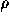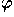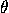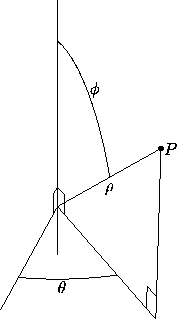# 9.3 Spherical Coordinates in Space

To define spherical coordinates, we take an axis (the polar axis) and a perpendicular plane (the equatorial plane), on which we choose a ray (the initial ray) originating at the intersection of the plane and the axis (the origin O). The coordinates of a point P are: the distancefrom P to the origin; the angle(zenith) between the line OP and the positive polar axis; and the angle(azimuth) between the initial ray and the projection of OP to the equatorial plane. See Figure 1. As in the case of polar and cylindrical coordinates,is only defined up to multiples of 360°, and likewise. Usuallyis assigned a value between 0 and 180°, but values ofbetween 180° and 360° can also be used; the triples (,,) and (, 360°-, 180°+) represent the same point. Similarly, one can extendto negative values; the triples (,,) and (-, 180°-, 180°+) represent the same point.Figure 1: A set of spherical coordinates for P is (,,)=(10,60°,30°).

Next: 9.4 Relations between Cartesian, Cylindrical, and Spherical Coordinates
Up: 9 Coordinate Systems in Space
Previous: 9.2 Cylindrical Coordinates in SpaceThe Geometry Center Home Page

Silvio Levy
Wed Oct 4 16:41:25 PDT 1995

This document is excerpted from the 30th Edition of the CRC Standard Mathematical Tables and Formulas (CRC Press). Unauthorized duplication is forbidden.